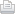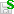https://doi.org/10.5194/hess-2019-283
https://doi.org/10.5194/hess-2019-283
09 Jul 2019
Status: this discussion paper is a preprint. It has been under review for the journal Hydrology and Earth System Sciences (HESS). The manuscript was not accepted for further review after discussion.

# Towards understanding the mean annual water-energy balance equation based on an Ohms-type approach

Xu Shan, Xingdong Li, and Hanbo Yang Xu Shan et al.
• State Key Laboratory of Hydro-Science and Engineering, Department of Hydraulic Engineering, Tsinghua University, Beijing 100084, China

Abstract. The Budyko hypothesis has been widely used to describe precipitation partitioning at the catchment scale. Many empirical and analytical formulas have been proposed to describe the Budyko hypothesis. Based on dimensional analysis and mathematic reasoning, previous studies gave an analytical derivation, i.e., the Mezentsev-Choudhury-Yang (MCY) equation. However, few hydrological processes were involved in the derivation. Therefore, this study firstly defines a catchment network to describe water vapor transformation and transportation using the Lagrangian particle tracking method; and then proposes the generalized flux of water vapor, which can be expressed as the ratio of potential difference with resistance. Furthermore, this study obtains a new constraint for the mean annual water-energy balance, 1 f(E)  =  1 f(E0)  +  1 f(P) with E, E0 and P being evaporation, potential evaporation and precipitation, respectively, and f( ) being a function of generalized flux, based on an analogy of the Ohms-type approach and the homogeneity assumption, i.e., the generalized flux has the same form for both water vapor transportation and chase transformation, and in other words, precipitation and potential evaporation have an equalized effect on evaporation. According to this constraint, the MCY equation can be obtained when the generalized flux f( ) is a power function. In addition, this study suggests a more general expression E  =  P(b+kE0) [pn+(b+kE0n]1/n under conditions without the homogeneity constraint, where E, E0 and P are evaporation, potential evaporation and precipitation, respectively, and n, k and b are constants (MCY equation when b = 0 and k = 1).

Share

Xu Shan et al.

Status: closed
Status: closed
AC: Author comment | RC: Referee comment | SC: Short comment | EC: Editor comment- Printer-friendly version- Supplement

Status: closed
Status: closed
AC: Author comment | RC: Referee comment | SC: Short comment | EC: Editor comment- Printer-friendly version- Supplement

Xu Shan et al.

Xu Shan et al.

## Viewed

Total article views: 1,308 (including HTML, PDF, and XML)
HTML PDF XML Total BibTeX EndNote
1,065 217 26 1,308 30 26
• HTML: 1,065
• PDF: 217
• XML: 26
• Total: 1,308
• BibTeX: 30
• EndNote: 26